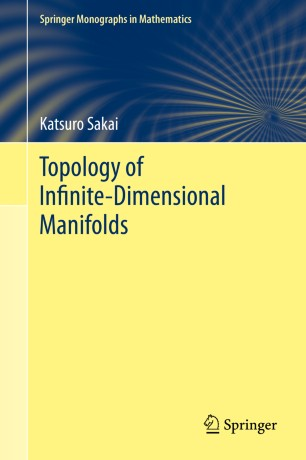# Topology of Infinite-Dimensional Manifolds

• Katsuro SakaiBook

Part of the Springer Monographs in Mathematics book series (SMM)

1. Front Matter
Pages i-xv
2. Katsuro Sakai
Pages 1-80
3. Katsuro Sakai
Pages 81-201
4. Katsuro Sakai
Pages 203-288
5. Katsuro Sakai
Pages 289-352
6. Katsuro Sakai
Pages 353-477
7. Katsuro Sakai
Pages 479-580
8. Back Matter
Pages 581-619

### Introduction

An infinite-dimensional manifold is a topological manifold modeled on some infinite-dimensional homogeneous space called a model space. In this book, the following spaces are considered model spaces: Hilbert space (or non-separable Hilbert spaces), the Hilbert cube, dense subspaces of Hilbert spaces being universal spaces for absolute Borel spaces, the direct limit of Euclidean spaces, and the direct limit of Hilbert cubes (which is homeomorphic to the dual of a separable infinite-dimensional Banach space with bounded weak-star topology).
This book is designed for graduate students to acquire knowledge of fundamental results on infinite-dimensional manifolds and their characterizations. To read and understand this book, some background is required even for senior graduate students in topology, but that background knowledge is minimized and is listed in the first chapter so that references can easily be found. Almost all necessary background information is found in Geometric Aspects of General Topology, the author's first book.
Many kinds of hyperspaces and function spaces are investigated in various branches of mathematics, which are mostly infinite-dimensional. Among them, many examples of infinite-dimensional manifolds have been found. For researchers studying such objects, this book will be very helpful. As outstanding applications of Hilbert cube manifolds, the book contains proofs of the topological invariance of Whitehead torsion and Borsuk’s conjecture on the homotopy type of compact ANRs. This is also the first book that presents combinatorial ∞-manifolds, the infinite-dimensional version of combinatorial n-manifolds, and proofs of two remarkable results, that is, any triangulation of each manifold modeled on the direct limit of Euclidean spaces is a combinatorial ∞-manifold and the Hauptvermutung for them is true.

### Keywords

Hilbert manifold (not necessary separable) Hilbert cube manifold Absorbing sets for absolute Borel classes The direct limit of Euclidean spaces (Hilbert cubes) Combinatorial infinite-dimensional manifold

#### Authors and affiliations

• Katsuro Sakai
• 1
1. 1.Department of Mathematics, Faculty of EngineeringKanagawa UniversityYokohamaJapan